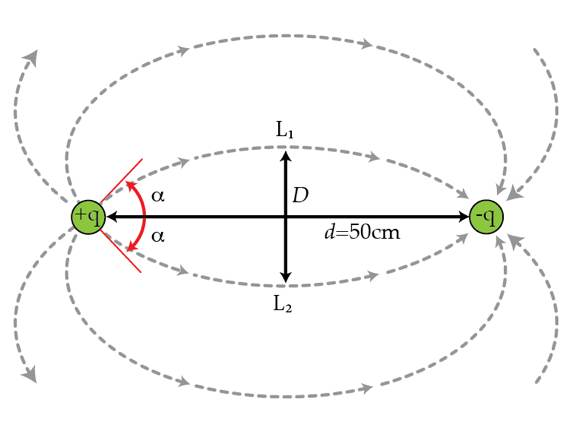Two charges $+q$ and $-q$ are separated by a distance $d=50~\mbox{cm}$. The electric field lines for this system form the the well-known pattern showed in the figure below. Consider two lines $L_{1}$ and $L_{2}$ lying on the same plane and leaving the positive charge at $\alpha=\pm 45^{\circ}.$ What is the maximum distance $D$ between the lines in centimeters.